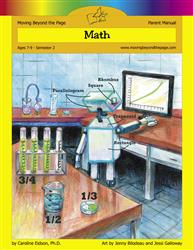# Common Core Alignment

CCSS.Math.Content.3.MD.8 - Solve real world and mathematical problems involving perimeters of polygons, including finding the perimeter given the side lengths, finding an unknown side length, and exhibiting rectangles with the same perimeter and different areas or with the same area and different perimeters.

## 5: MathUnit 5: Area and Perimeter
Lesson 10: Problem Solving With Perimeter and Area